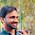This is a simple C# program code to find area of a rectangle, circle and triangle with user interface and example.

Manually we can find the area of a rectangle by using equation:

• Area = Breadth * Length.
Also we can find the area of a circle by using equation:

• Area = 3.14 * (radius)2

Also we can find the area of a triangle by using equation:

a = length of first side
b = length of second side
c = length of third side
s = (a + b+ c)/2

• Area = sqrt(s*(s-a)*(s-b)*(s-c))

-where sqrt stands for square root

User interface with exampleHere ‘Find Area’ is the ‘button_1’. There are three tabs: One is for ‘Rectangle’, another one is for ‘Triangle’ and last is for ‘Circle’.

Code Behind interface:

 using System; using System.Collections.Generic; using System.ComponentModel; using System.Data; using System.Drawing; using System.Linq; using System.Text; using System.Windows.Forms; namespace area {     public partial class Form1 : Form     {         public Form1()         {             InitializeComponent();         }         private void button1_Click(object sender, EventArgs e)         {             if (tabControl1.SelectedTab == tabPage1)             {                 double breadth = Convert.ToDouble(textBox1.Text);                 double lenght = Convert.ToDouble(textBox2.Text);                 label5.Text = (breadth * lenght).ToString();             }             if (tabControl1.SelectedTab == tabPage2)             {                 double a = Convert.ToDouble(textBox4.Text);                 double b = Convert.ToDouble(textBox5.Text);                 double c = Convert.ToDouble(textBox6.Text);                 double s = (a + b + c) / 2;                 double Area = Math.Sqrt(s * (s - a) * (s - b) * (s - c));                 label5.Text = (Area).ToString();             }             if (tabControl1.SelectedTab == tabPage3)             {                 double  radius = Convert.ToDouble(textBox3.Text);                 label5.Text = (3.14 * radius).ToString();             }         }     } }

1.the code is good ,but i need the code without using tabs as per my requirement i need code by using textbox,button and list items (or) label can u make the code for it

2.Instead of tabs, You can use listbox. Then add 3 items to listbox, named as 'Rectangle', Triangle' and 'Circle'.
Now instead of tab selection code( if (tabControl1.SelectedTab == tabPage1) ) use list box control line:
if(listbox1.text =="Rectangle")
{
// Code for finding area of rectangle
}

Like this, write code for finding area of triangle and circle